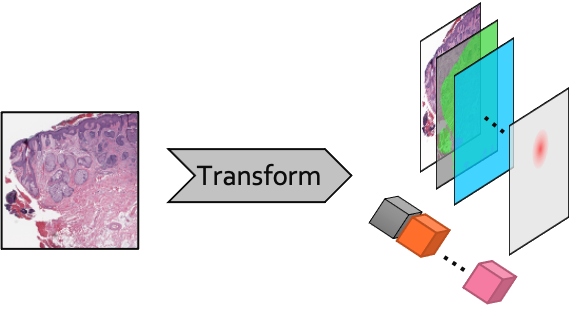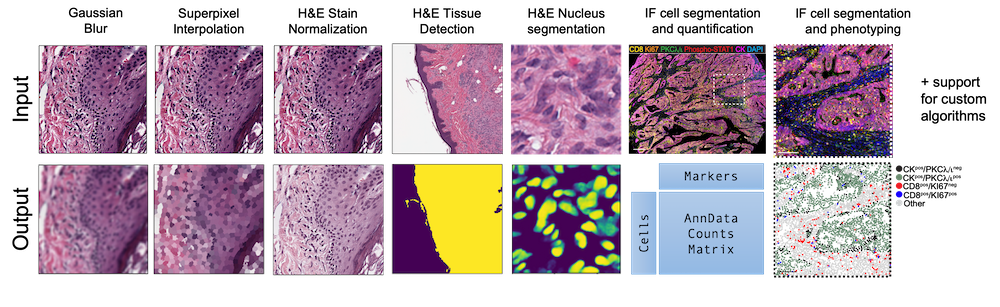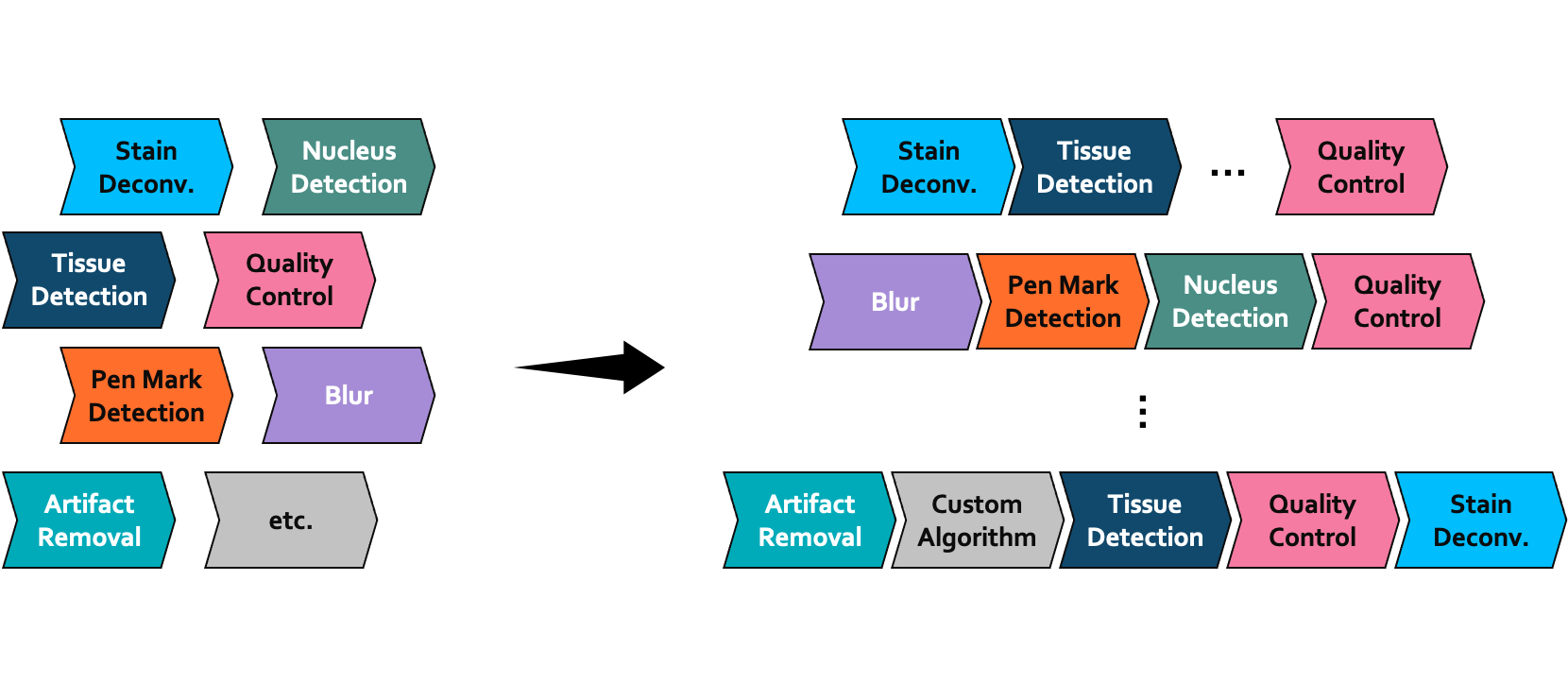# Creating Preprocessing Pipelines

Preprocessing pipelines define how raw images are transformed and prepared for downstream analysis. The `pathml.preprocessing` module provides tools to define modular preprocessing pipelines for whole-slide images.

In this section we will walk through how to define a `Pipeline` object by composing pre-made `Transform` objects, and how to implement a new custom `Transform`.

## What is a Transform?

The `Transform` is the building block for creating preprocessing pipelines.

Each `Transform` applies a specific operation to a `Tile` which may include modifying an input image, creating or modifying pixel-level metadata (i.e., masks), or creating or modifying image-level metadata (e.g., image quality metrics or an AnnData counts matrix).Schematic diagram of a Transform operating on a tile. In this example, several masks are created (represented by stacked rectangles) as well as several labels (depicted here as cubes).Examples of several types of Transform

## What is a Pipeline?

A preprocessing pipeline is a set of independent operations applied sequentially. In `PathML`, a `Pipeline` is defined as a sequence of `Transform` objects. This makes it easy to compose a custom `Pipeline` by mixing-and-matching:Schematic diagram of Pipeline composition from a set of modular components

In the PathML API, this is concise:

```from pathml.preprocessing import Pipeline, BoxBlur, TissueDetectionHE

pipeline = Pipeline([
BoxBlur(kernel_size=15),
threshold=30, outer_contours_only=True)
])
```

In this example, the preprocessing pipeline will first apply a box blur kernel, and then apply tissue detection.

## Creating custom Transforms

Note

In some cases, you may want to implement a custom `Transform`. For example, you may want to apply a transformation which is not already implemented in `PathML`. Or, perhaps you want to create a new transformation which combines several others.

To define a new custom `Transform`, all you need to do is create a class which inherits from `Transform` and implements an `apply()` method which takes a `Tile` as an argument and modifies it in place. You may also implement a functional method `F()`, although that is not strictly required.

For example, let’s take a look at how `BoxBlur` is implemented:

```class BoxBlur(Transform):
"""Box (average) blur kernel."""
def __init__(self, kernel_size=5):
self.kernel_size = kernel_size

def F(self, image):
return cv2.boxFilter(image, ksize = (self.kernel_size, self.kernel_size), ddepth = -1)

def apply(self, tile):
tile.image = self.F(tile.image)
```

Once you define your custom `Transform`, you can plug it in with any of the other `Pipeline`, etc.